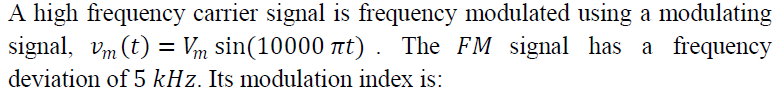1. The use of distributed computing facilities for application requiring large computing power over a long period of time is:

(a) High-Performance Computing
(b) High-Throughput Computing
(d) Distributed Computing

2. A small change in either the plaintext or the key producing a significant change in the ciphertext is called:

(a) Feistel effect
(b) Claude Shannon effect
(c) Bit independence effect
(d) Avalanche effect

3. Finding an effective linear equation between plaintext, ciphertext and the key that holds with probability 𝑝 ≠0.5 is the objective of:

(a) Differential Cryptanalysis
(b) Linear Cryptanalysis
(c) Brute-Force Attack
(d) Feistel Analysis

4. During the design of 𝑆 -boxes used in various cryptographic algorithms, if for a 1-bit input change, at least 𝜋 output bits change, then it satisfies:

(a) Strict avalanche criterion
(b) Bit independence criterion
(c) Permutation criterion
(d) Guaranteed avalanche criterion

5. The block cipher mode of operation used for encryption of stream-oriented transmission over noisy channel is:

(a) Electronic Codebook
(b) Output Feedback
(c) Cipher Feedback
(d) Cipher Block Chaining

6. A popular approach to generating a secure pseudorandom number is known as:

(a) Pseudorandom Number Generator (PRNG)
(b) Linear Congruential Generator (LCG)
(c) True Random Number Generator (TRNG)
(d) Blum, Blum, Shub Generator (BBS)

7. Which Novell NetWare Utility Program allows one to recover deleted files from network drives only?

(a) Map
(b) Salvage
(c) Filter
(d) Capture

8. What type of problem can be caused by the electromagnetic field of speakers?

(a) Distortion of video display
(b) RAM errors
(c) Computer shut down
(d) Read / write problem on magnetic disks and tapes

9. In a 100 % modulated AM signal with carrier power 100 𝑊, the power in the upper sideband is:

(a) 75 𝑊
(b) 66 𝑊
(c) 50 𝑊
(d) 25 𝑊